Select Page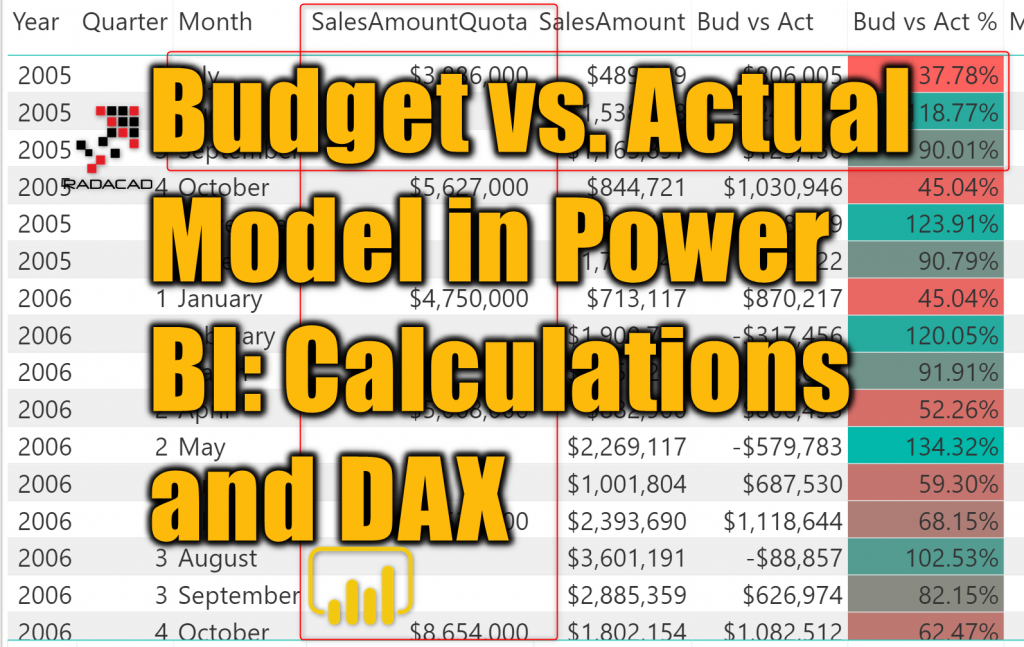In the previous article, I explained how you can build a data model for budget vs. actual, where the grain of the two fact tables is different. The model works perfectly as a star schema. As long as you slice and dice data in the level of granularity that both tables support, then you don’t need anything else. However, if you want to go to lower grain than what the table supports, then you would need more calculations, and DAX can handle that easily. In this article, I’m going to explain some calculations using DAX that helps to go to a lower grain that what the fact table supports. To learn more about Power BI, read Power BI book from Rookie to Rock Star.

Prerequisite

I explained in this article, how to build a Budget vs. Actual Zero Complexity data model in Power BI.

There is no prerequisite for this article. However, I highly recommend you to read below articles beforehand;

Sample Dataset

The sample dataset used here is the AdventureWorksDW Excel file which can be downloaded from here.

The Model

The model that we build for this sample in the previous article is as below: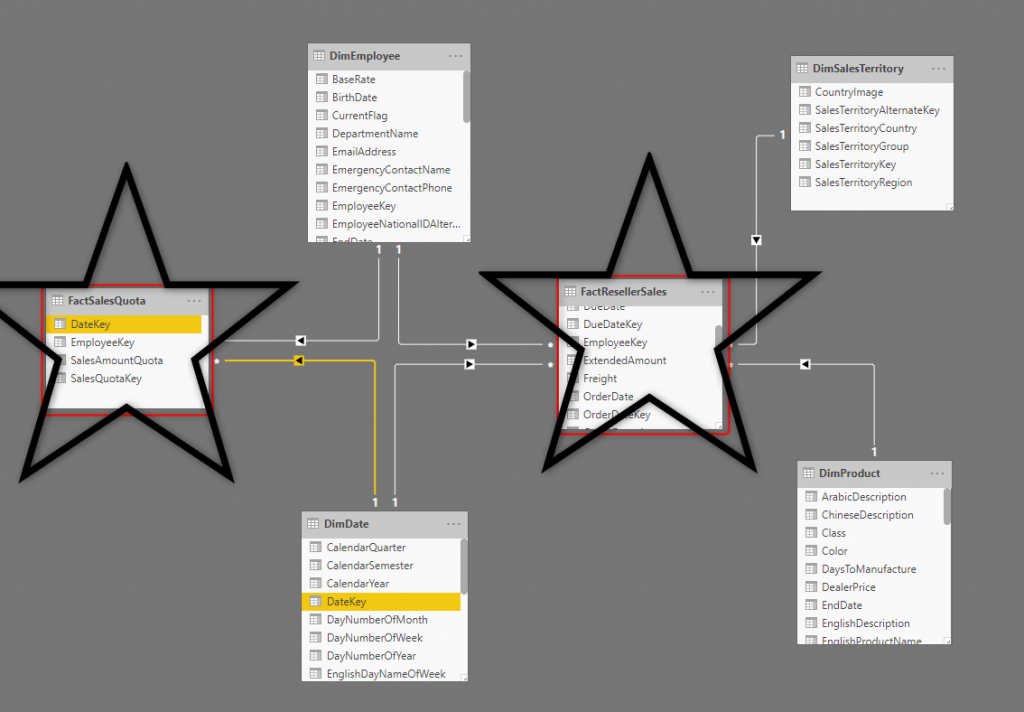In the data model above, we have two fact tables;

• FactResellerSales which is on a grain of every DAY, every product, SalesTerritory, and Employee.
• FactSalesQuota which is on a grain of every QUARTER, and Employee

To connect both tables to the same Date dimension, we considered the first day of every quarter as the Date field for the FactSalesQuota, and then connected them through a star schema approach.

Here is how the data in the Sales Quota table looks like: (FactSalesQuota in the AdventureWorksDW)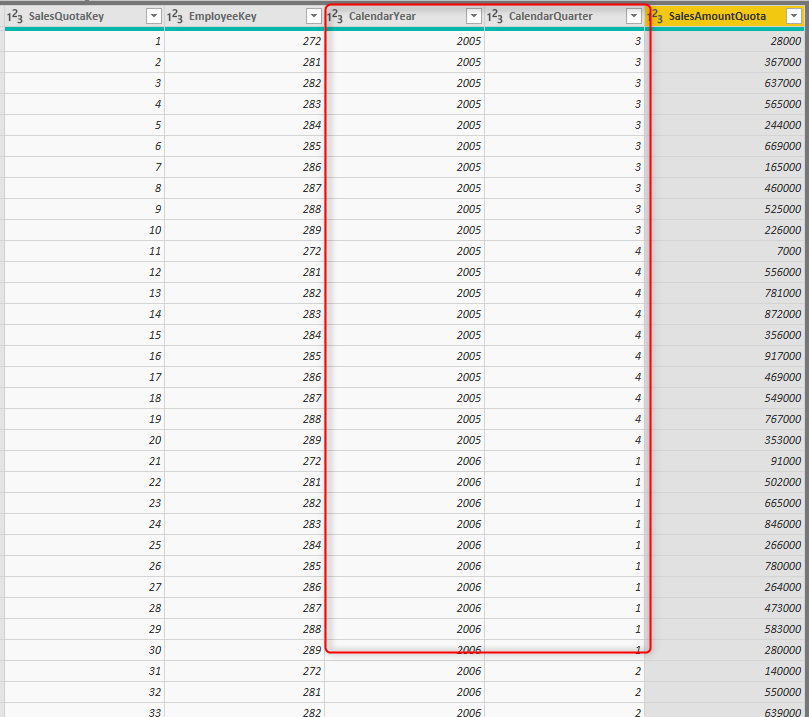Lower Granularity Problem

The schema above works perfectly if you slice and dice data by the grain supported by the budget table (or let’s say FactSalesQuota table) which is Quarter in this example. However, if you start analyzing data on a lower grain, for example, Month, you get something like this: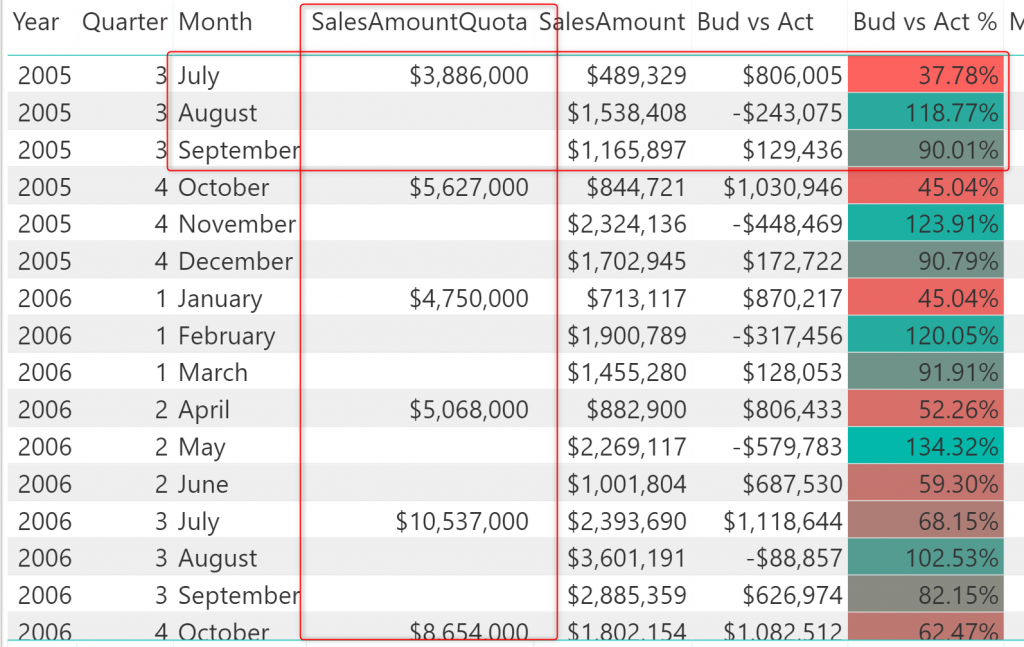As you can see the FactSalesQuota only have values in the first month of each quarter. In a monthly analysis of the data, the FactResellerSales has values for each month, but because the other table doesn’t, then the calculation of budget vs. actual would be wrong in every month, for the first month, the budget value is so high (because it is for 3 months), and for the other two months is blank.

The important note here is that the budget data is only valid down to quarter level. Because there is no monthly budget, so the monthly analysis of that doesn’t make sense. However, sometimes, the requirement is, to calculate the budget split of the quarterly budget into monthly, weekly, or even daily. And that is what we are going to explore in this article.

Calculate Monthly from the Quarterly

The monthly calculation seems to be simple, each quarter will be three months in total, so the calculation of that would be the quarterly value divided by three. However, the problem is that when you go to the monthly level of slicing and dicing, then for some months, there is no value!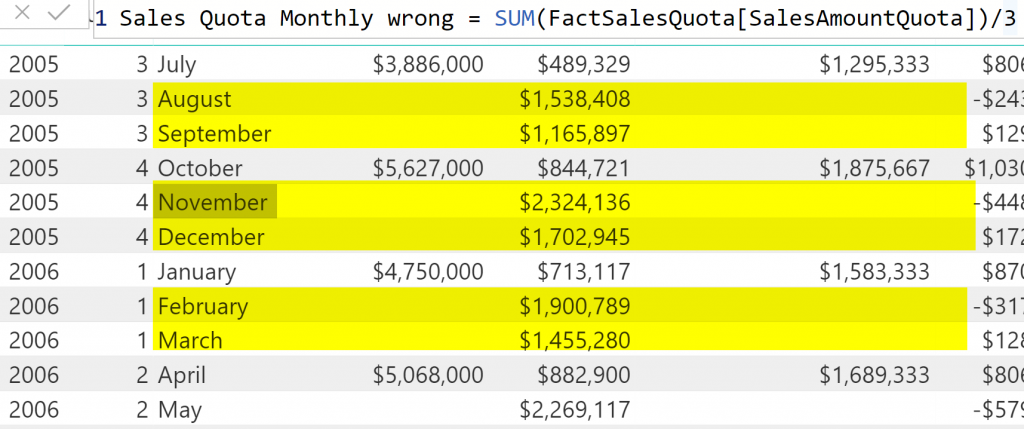You should use some of the DAX techniques to overcome the issue. The issue is that in a given month, there might be no SalesQuota. Let’s go through an example: for August 2005: there is no SalesQuota for this month because the SalesQuota is for the 1st of July 2005. So, your job, in the DAX calculation, is to get the first quarter of each month (if your budget data is monthly, then you should get the first of each month instead). and then get the SalesQuota of that date.

I used LASTDATE function to find out the current date in the visual’s row, and then STARTOFQUARTER function to fetch the start of that quarter. Finally, I wrapped it all inside a CALCULATE function to get the SalesQuota for the first of the quarter and then divided it by three to get the monthly Sales Quota. Here is the calculation: